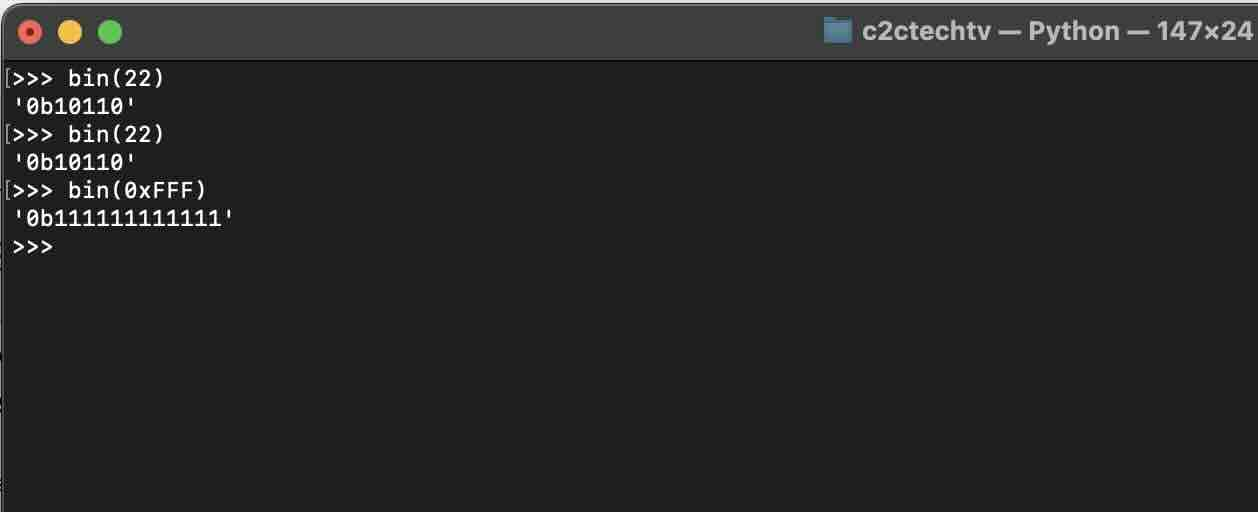# Python: Convert int to binary String

We can easily convert int in Python to binary string using the built-in bin() function.

bin(): convert an integer number to a binary string prefixed with “0b”

Documentation: https://docs.python.org/3/library/functions.html#bin

Let's take a look at a few examples.

## Example 1: decimal int to binary string

``````decimal_number = 42
binary_str = bin(decimal_number)
print(f"Binary of {decimal_number} =  {binary_str}")
``````
Output:

Binary of 42 = 0b101010

## Example 2: hexadecimal int to binary string

``````hex_number = "FEFE"
binary_str = bin(int(hex_number, 16))
print(f"Binary of {hex_number}: {binary_str}")``````
Output:

Binary of FEFE: 0b1111111011111110

## Examples using Python interactive Shell-

### Facing issues? Have Questions? Post them here! I am happy to answer!

Author Info:

Rakesh (He/Him) is a seasoned developer with over 10 years of experience in web and app development, and a deep knowledge of operating systems. Author of insightful How-To articles for Code2care.